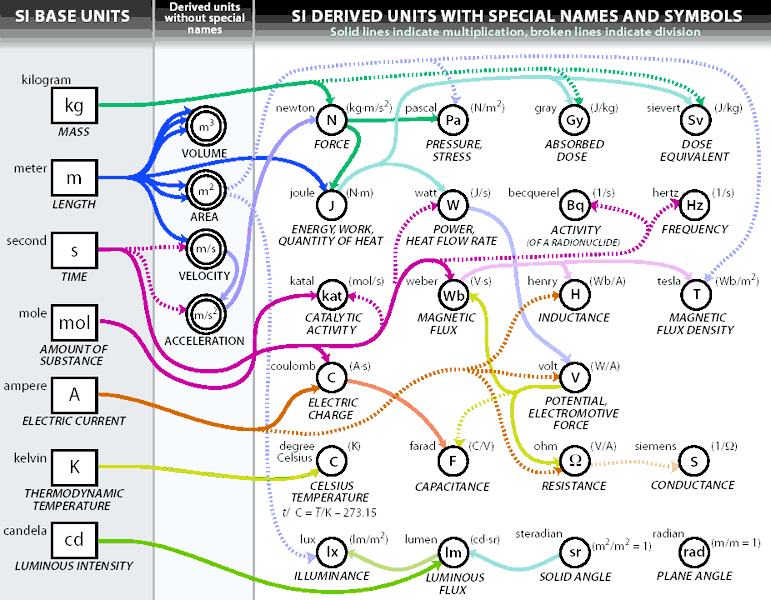# EquivalentsThe diagram above shows graphically how the 22 SI derived units with special names and symbols are related to the seven SI base units. In the first column, the symbols of the SI base units are shown in rectangles, with the name of the unit shown toward the upper left of the rectangle and the name of the associated base quantity shown in italic type below the rectangle. In the third column the symbols of the derived units with special names are shown in solid circles, with the name of the unit shown toward the upper left of the circle, the name of the associated derived quantity shown in italic type below the circle, and an expression for the derived unit in terms of other units shown toward the upper right in parenthesis. In the second column are shown those derived units without special names [the cubic meter (m3) excepted] that are used in the derivation of the derived units with special names. In the diagram, the derivation of each derived unit is indicated by arrows that bring in units in the numerator (solid lines) and units in the denominator (broken lines), as appropriate.

Two SI derived units with special names and symbols, the radian, symbol rad, and the steradian, symbol sr (bottom of the third column of the diagram), are shown without any connections to SI base units – either direct or through other SI derived units. The reason is that in the SI, the quantities plane angle and solid angle are defined in such a way that their dimension is one – they are so-called dimensionless quantities. This means that the coherent SI derived unit for each of these quantities is the number one, symbol 1. That is, because plane angle is expressed as the ratio of two lengths, and solid angle as the ratio of an area and the square of a length, the SI derived unit for plane angle is m/m = 1, and the SI derived unit for solid angle is m2/m2 = 1. To aid understanding, the special name radian with symbol rad is given to the number 1 for use in expressing values of plane angle; and the special name steradian with symbol sr is given to the number 1 for use in expressing values of solid angle. However, one has the option of using or not using these names and symbols in expressions for other SI derived units, as is convenient.

The unit "degree Celsius" which is equal to the unit "Kelvin" is used to express Celsius temperature T. In this case, “degree Celsius" is a special name used in place of "kelvin" This equality is indicated in the diagram by the symbol K in parenthesis toward the upper right of the oC circle. The equation below "CELSIUS TEMPERATURE" relates Celsius temperature t to thermodynamic temperature T. An interval or difference of Celsius temperature can, however, be expressed in kelvins as well as in degrees Celsius.